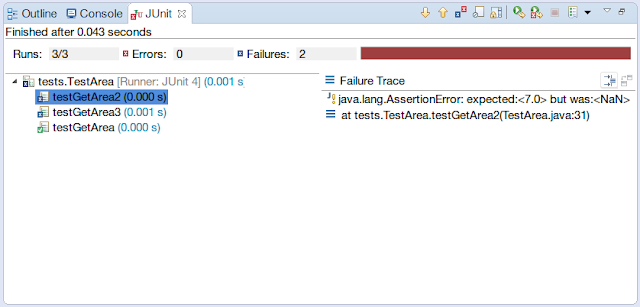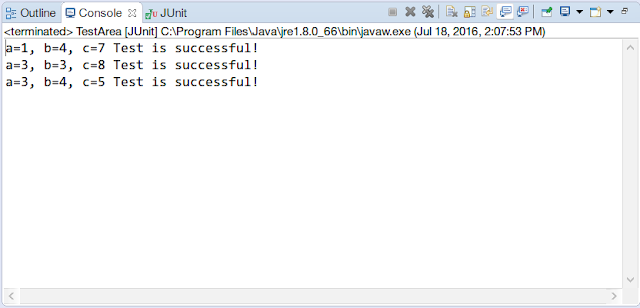Hi everyone,

### In this tutorial, I am going to explain an important topic in software development called Testing and going to give information about Unit Testing in Java Programming Language (JUnit).

Testing is the process of checking the functionality of the developed code/program to find bugs/errors. There are mainly 2 types of testing: Manual Testing and Automated Testing

In Manual Testing, the testing is done manually by the developer/tester without using any automated tools. There are different stages for manual testing such as unit testing, integration testing, system testing, and user acceptance testing.

On the other hand, in Automated Testing, the tester writes scripts and uses another software to test the product. Regression testing and Unit testing can be given as examples to Automated Testing. Apart from regression testing, automation testing is also used to test the application from load, performance, and stress point of views.

One of the most important testing methods is called Unit Testing and it is essential to every software company to provide a quality product to their customers. If we want to summarize Unit Testing in a few words, we can say that Unit Testing is the testing of a single entity (class or method).

### What is JUnit?

JUnit is a unit testing framework specifically for the Java Programming Language and it is very important for the Test Driven Development.

JUnit suggests the idea of "first testing then coding", which lays emphasis on setting up the test data for a piece of code which can be tested first and then can be implemented . This approach increases the productivity of the programmers and reduces the debugging time.

• JUnit tests can be run automatically and they check their own results and provide immediate feedback.
• JUnit tests can be organized into test suites containing test cases and even other test suites.
• JUnit shows test progress in a bar that is green if test is successful and it turns red when a test fails.
• The JUnit framework is annotation based. Let's see the annotations that can be used while writing tests:

### Annotations for Junit testing

@Test annotation specifies that method is the test method.

@Test(timeout=1000) annotation specifies that method will be failed if it takes longer than 1000 milliseconds (1 second).

@BeforeClass annotation specifies that method will be invoked only once, before starting all the tests.

@Before annotation specifies that method will be invoked before each test.

@After annotation specifies that method will be invoked after each test.

@AfterClass annotation specifies that method will be invoked only once, after finishing all the tests.

### Requirements of JUnit Testing:

First step: Select a good IDE(Eclipse, IntelliJ..etc.) for Java Development.

Second step: Install JUnit from this website. (Unless you are using Eclipse or IntelliJ. JUnit is already integrated in these advanced IDEs.)

Third step: Create your test classes and run them to check for any kinds of errors in your codes.

### How to define a test in JUnit?

A single JUnit test is a method contained in a class which is only used for testing. This is called a Test class. To define a method as a unit test, you annotate it with the @Test annotation.

This method is responsible for providing the test code. You use an assert method, provided by JUnit or another assert framework, to check an expected result versus the actual result. These method calls are typically called asserts. In order to easily identify the problems in your code, you should provide meaningful messages in assert statements. This is especially important if someone else wrote the code and you are testing it.

### Assert class

The Assert class provides methods to assert the program logic.

The common methods of Assert class are as follows:

void assertEquals(boolean expect,boolean actual): checks if the two primitives/objects are equal.
void assertTrue(boolean condition): checks if the condition is true.
void assertFalse(boolean condition): checks if the condition is false.
void assertNull(Object obj): checks if the object is null.
void assertNotNull(Object obj): checks if the object is not null.

After introducing the JUnit and its features let's have a look at an example test in Eclipse IDE:

### An Example Test:

First let's create a new project and then create our class Area in a package called herrberk. This class basically calculates the area of a triangle using Heron's Formula.

And then, in another package called tests, let's create a new test class called TestArea. It is not obligatory to name our test classes as Test****, but it is a good practice to be able to avoid confusions.

In our test class, create a method called public void testGetArea(), add @Test annotation right above the method and click on the "Add JUnit 4 library to the build path" as shown in the following figure.

After this you will notice that the JUnit 4 library will appear in your project directory as shown below;

``````
package herrberk;

public class Area {

//Calculates the Area of a Triangle
public static double getArea(double a, double b, double c) {
double s = (a + b + c)/2.0; //s = perimeter/2
double x = ((s) * (s-a) * (s-b) * (s-c)); //herons formula
double area = Math.sqrt(x);

return area;
}
}

``````

Now let's create the test for this code;

``````
package tests;

import herrberk.Area;
import org.junit.Test;
import static org.junit.Assert.*;

public class TestArea {

// DELTA is used as a threshold
// if the difference between the expected and the returned
// is less than this delta then the test returns true.
private static final double DELTA = 1e-15;

@Test
public void testGetArea(){
double a = 3;
double b = 4;
double c = 5;
assertEquals(6.0 ,Area.getArea(a, b, c), DELTA);
System.out.println("a=3, b=4, c=5 Test is successful!");
}

@Test
public void testGetArea2(){
double a = 1;
double b = 4;  // This can not be a triangle!
double c = 7;
// The area will be NaN not 7.0 ! This is not a triangle!
// Test will not pass!
assertEquals(7.0 , Area.getArea(a, b, c), DELTA);
System.out.println("a=1, b=4, c=7 Test is successful!");
}
@Test
public void testGetArea3(){
double a = 3;
double b = 3;  // This can not be a triangle!
double c = 8;
// The area will be NaN not 7.0 ! This is not a triangle!
// Test will not pass!
assertEquals(9.0 , Area.getArea(a, b, c), DELTA);
System.out.println("a=3, b=3, c=8 Test is successful!");

}
}

``````
If we run the tests, we will see 1 pass, 2 fails. The reason behind this is in the testGetArea2 and testGetArea3 tests we expected the areas to be nonzero but actually these sides cannot form a triangle!  and the areas are NaN (Not a Number).And after seeing this test result we should fix the getArea class to check if the triangle is a valid triangle and edit our test cases as follows.

``````
package herrberk;

public class Area {

// Calculates the Area of a Triangle
public static double getArea(double a, double b, double c) {
double s = (a + b + c) / 2.0; // s = perimeter/2
// If we can not form a triangle return 0.0
if (a + b <= c || b + c <= a || a + c <= b) {
return 0.0;
} else {
double x = ((s) * (s - a) * (s - b) * (s - c)); // herons formula
double area = Math.sqrt(x);
return area;
}
}
}

``````

``````
package tests;

import herrberk.Area;
import org.junit.Test;

import static org.junit.Assert.*;

public class TestArea {

// DELTA is used as a threshold
// if the difference between the expected and the returned
// is less than this delta then the test returns true.
private static final double DELTA = 1e-15;

@Test
public void testGetArea(){
double a = 3;
double b = 4;
double c = 5;
assertEquals(6.0 ,Area.getArea(a, b, c), DELTA);
System.out.println("a=3, b=4, c=5 Test is successful!");
}

@Test
public void testGetArea2(){
double a = 1;
double b = 4;  // This can not be a triangle!The area is 0.0
double c = 7;
// The area will be 0.0 not 7.0 ! This is not a triangle!
assertEquals(0.0 , Area.getArea(a, b, c), DELTA);
System.out.println("a=1, b=4, c=7 Test is successful!");
}

@Test
public void testGetArea3(){
double a = 3;
double b = 3;  // This can not be a triangle again! The area is 0.0
double c = 8;
assertEquals(0.0 , Area.getArea(a, b, c), DELTA);
System.out.println("a=3, b=3, c=8 Test is successful!");
}
}

``````
Here is the result after the edits:As you can see, all the tests have passed and we fixed a problem in our code! I hope you can see the importance of testing during the development process from this simple example. Always, whenever you write your codes, try to write test cases and test the functionality of your code to avoid unexpected behavior in the future!

That's all for this tutorial. I hope all of your tests stay green! Happy Coding!